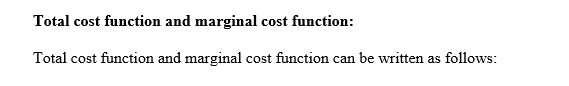# This question asks you to find the socially optimal outcome. Suppose a social planner takes the demand function and cost functions as given, and can choose (i) the market price, (ii) the number of firms, and (iii) the quantity produced by each firm [the planner is free to choose any quantity, even if the firms would lose money]. The social planner’sgoal is to maximize the sum of consumer surplus and firms’ profits. To do this, the planner will take into account the two economic principles of efficiency, (i) Allocative efficiency which implies price=mc. (2) Efficiency in production, which means that the cost of production of the total market quantity should be minimized. Suppose the demand function is Q(p) = 40 – 5p. For each of the following cost functions, what market price, number of firms and quantity for each firm will the social planner choose?a) C(q) = 20 + 4qb) C(q) = 3 + q^2/3 + 3q.

Question
3 views

This question asks you to find the socially optimal outcome. Suppose a social planner takes the demand function and cost functions as given, and can choose (i) the market price, (ii) the number of firms, and (iii) the quantity produced by each firm [the planner is free to choose any quantity, even if the firms would lose money]. The social planner’s
goal is to maximize the sum of consumer surplus and firms’ profits. To do this, the planner will take into account the two economic principles of efficiency, (i) Allocative efficiency which implies price=mc. (2) Efficiency in production, which means that the cost of production of the total market quantity should be minimized. Suppose the demand function is Q(p) = 40 – 5p. For each of the following cost functions, what market price, number of firms and quantity for each firm will the social planner choose?

a) C(q) = 20 + 4q
b) C(q) = 3 + q^2/3 + 3q.

check_circle

Step 1

The given information:

Step 2

a.)

Inverse demand function:

Inverse demand function can be written as follows

Step 3...

### Want to see the full answer?

See Solution

#### Want to see this answer and more?

Solutions are written by subject experts who are available 24/7. Questions are typically answered within 1 hour.*

See Solution
*Response times may vary by subject and question.
Tagged in